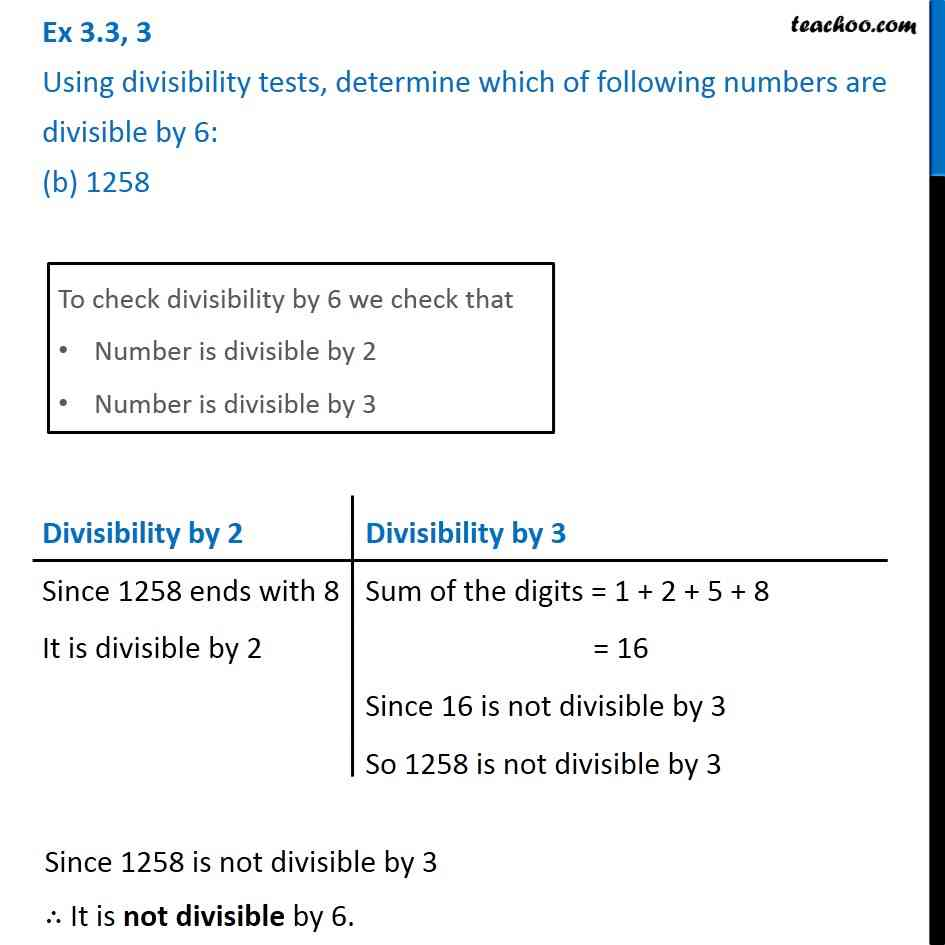1. Chapter 3 Class 6 Playing with Numbers
2. Serial order wise
3. Ex 3.3

Transcript

Ex 3.3, 3 Using divisibility tests, determine which of following numbers are divisible by 6: (b) 1258 To check divisibility by 6 we check that Number is divisible by 2 Number is divisible by 3 Divisibility by 2 Since 1258 ends with 8 It is divisible by 2 Divisibility by 3 Sum of the digits = 1 + 2 + 5 + 8 = 16 Since 16 is not divisible by 3 So 1258 is not divisible by 3 Since 1258 is not divisible by 3 ∴ It is not divisible by 6.